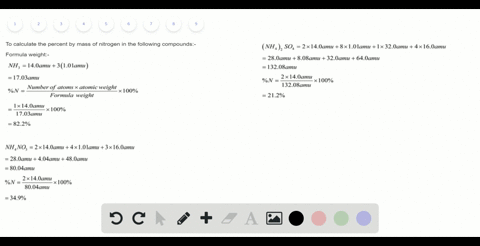Sign up for our free STEM online summer camps starting June 1st!View Summer Courses### The effectiveness of nitrogen fertilizers depends…

02:40Auburn University Main Campus

Need more help? Fill out this quick form to get professional live tutoring.

Get live tutoring
Problem 88

If $1.5 \mathrm{mol} \mathrm{C}_{2} \mathrm{H}_{5} \mathrm{OH}, 1.5 \mathrm{mol} \mathrm{C}_{3} \mathrm{H}_{8},$ and 1.5 $\mathrm{mol} \mathrm{CH}_{3} \mathrm{CH}_{2} \mathrm{COCH}_{3}$ are completely combusted in oxygen, which produces the largest number of moles of $\mathrm{H}_{2} \mathrm{O} ?$ Which produces the least? Explain.

So, largest number of moles of $\mathrm{H}_{2} \mathrm{O}$ is produced from $\mathrm{C}_{3} \mathrm{H}_{8}, \mathrm{CH}_{3} \mathrm{CH}_{2} \mathrm{COCH}_{3}$ and least
number of moles of $\mathrm{H}_{2} \mathrm{O}$ produced from $\mathrm{C}_{2} \mathrm{H}_{5} \mathrm{OH}.$

## Discussion

You must be signed in to discuss.

## Video Transcript

a way to solve this problem. First, you have to write down the balance equation for each compassion reaction. So the first one is set which five wage reacting with the oxygen to farm. Syrian is Jules. So because liquid form plus, I'm just directing writing the balanced equations here to save some time. So in desperation, relayed a three ox is, um and they will give us to cover the side and treat each tool. So this's the menace equation for the compassion of situation wage. Then we write down our second one, which is propane. Sit ridge eight Reacting with it. Five oxygen to give us three carbon dioxide class for water Water's We've been guess his firm in the compassion reactions. Uh, and the last one we have is since this is tow seal C is three. Witness it will give us a tea or two and ate his tool. Okay, Now we have our three balanced equation here, and from here we'LL have to find out how many moles off water we confined from the balanced equations here. So here you can see that one more off. See courage. Five ways will give us tree. Matloff it's, too, Um, the second one. One morning off, see through in change will give us formal off is to him. And in the final one, we can say, um, to moon off ceases to Susie's will give us eight more fish tour that men's one mole off. This will give us form all ofthe age, too. Now if we're given one point five mill off each wrecked and how many more off its tool will be produced. So if hey one one of citrus five, which give us three matloff h two o, that means one planned five months of this will give us three times one, but fine that men's four point five mill office tour and the second one, his formal of Cities Shade, is giving us formal statements one point five more. Well, give us four times one point five more net mints. Six more no face and a turtle in again, Since this is also formal statements one planned but five. Molly, give us six small no face to him. So from these three, we can see that, um, six months is the highest following among these three for a strong team is four point five second, one six tired and also six. So that man's ah, the largest number off mole off each two is produced in case of's history. Sees two CEOs his three and in case ofthe C three shades and the list number is produced in case of Sito Age five wage, which is four point five mill.